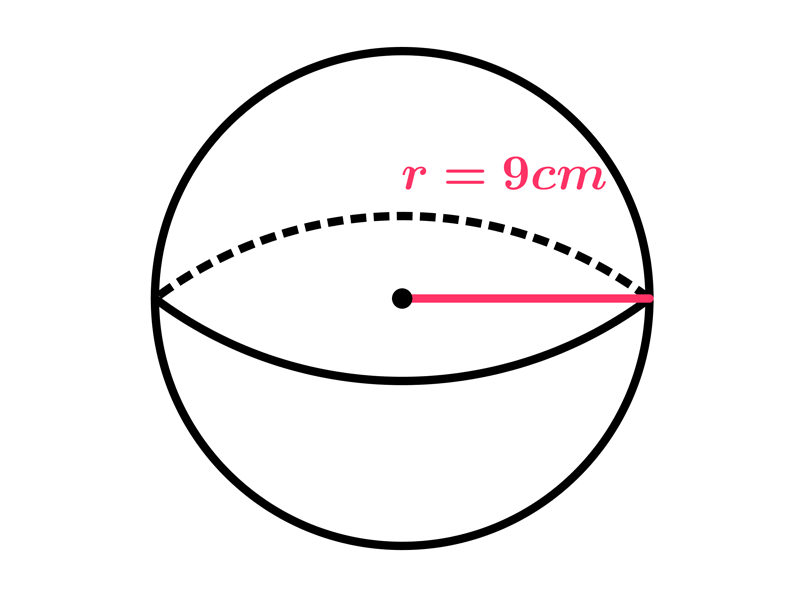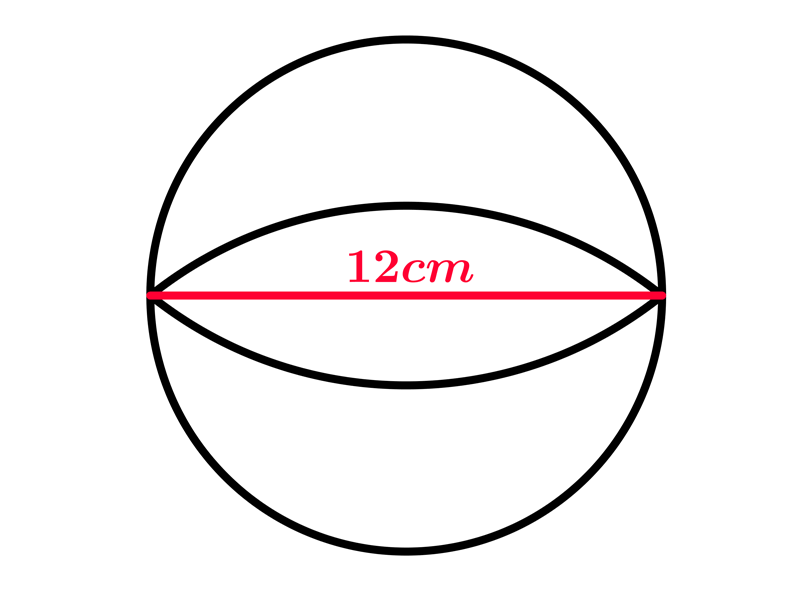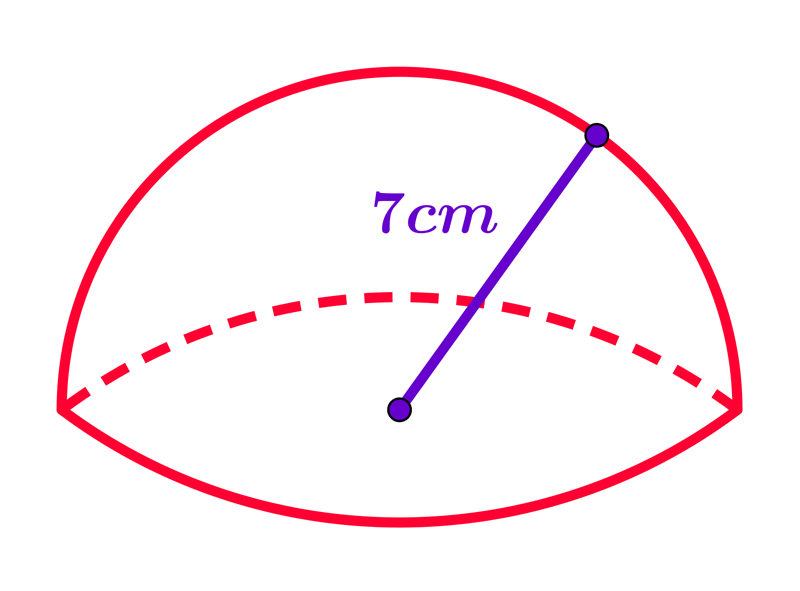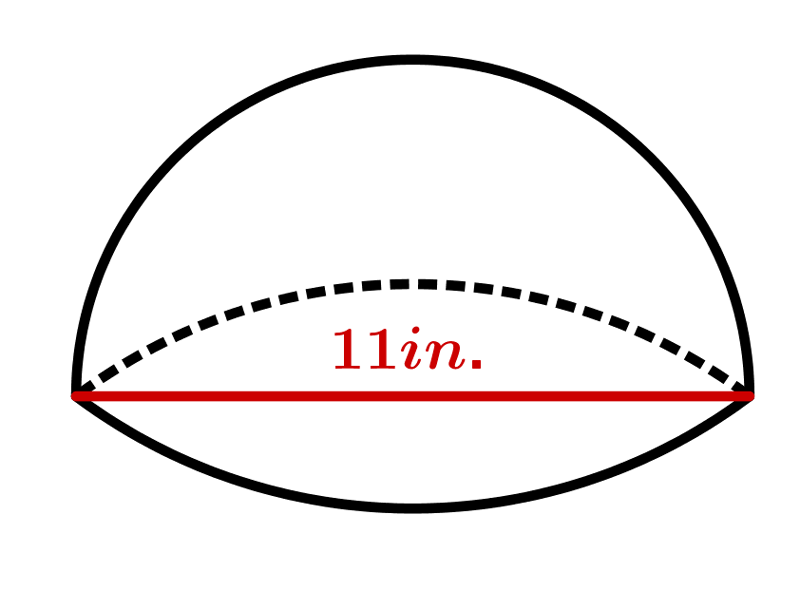Math Calculators, Lessons and Formulas

It is time to solve your math problem

mathportal.org
• Geometry
• Sphere

# Sphere

ans:
syntax error
C
DEL
ANS
±
(
)
÷
×
7
8
9
4
5
6
+
1
2
3
=
0
.
auto next question
calculator
•  Question 1: 1 pts Which expression can be used to find the volume of the sphere?
 $V=4R^{2}\pi$ $V=\dfrac{4}{3}R^{3}\pi$ $V=\dfrac{4}{3}R^{2}\pi$ $V=\dfrac{1}{3}R^{2}\pi$
•  Question 2: 1 pts The following expression can be used to find the total surface area of the sphere. $$A=4R^{2}\pi$$
•  Question 3: 1 pts Find the volume of a sphere with a radius of $3 cm.$
$V=$
•  Question 4: 1 pts Find the total surface area of the sphere with a radius of $7 cm.$
 $V=108\pi in. ^{2}$ $V=169\pi cm ^{2}$ $V=196\pi cm ^{2}$
•  Question 5: 2 pts Find the total surface area of the sphere shown on the picture.$A=144\pi cm ^{2}$ $A=324\pi cm ^{2}$ $A=224\pi cm ^{2}$
•  Question 6: 1 pts Find the volume of a sphere shown on the picture.$V=$
•  Question 7: 2 pts Which of the following expression can be used to find the total surface area of the hemisphere shown on the picture?$A=3\cdot 7^{2}\pi cm^{2}$ $A=2\cdot 7^{2}\pi cm^{2}$ $A=\dfrac{4}{3}\cdot 7^{2}\pi cm^{2}$ $A=3\cdot 7^{3}\pi cm^{2}$
•  Question 8: 2 pts Which of the following expression can be used to find the volume of the sphere shown on the picture?$V=$
•  Question 9: 3 pts Basketballs used in professional games must have a circumference of 81 centimeters. What is the surface area of a basketball used in a professional game?
 $A=\pi\dfrac{81^{2}}{4\cdot \pi^{2}}$ $A=4\pi\dfrac{81^{2}}{4\cdot \pi^{2}}$ $A=2\pi\dfrac{81^{2}}{4\cdot \pi}$ $A=4\pi\dfrac{81^{2}}{ \pi^{2}}$
•  Question 10: 3 pts We can use the following expression to find a ratio comparing the volume of a sphere with radius $r$ to the volume of a cylinder with radius$r$ and height $2r.$ $$V_{sphere}:V_{cylinder}=\dfrac{\dfrac{4}{3}r^{3}\pi}{\pi r^{2} \cdot 2r}=\dfrac{2}{3}$$
•  Question 11: 3 pts If the area of the great circle of a sphere is $32m^{2}$,what is the surface area of the sphere?
 $A=64\pi m^{2}$ $A=128 m^{2}$ $A=128\pi^{2} m^{2}$ $A=256\pi^{2} m^{2}$
•  Question 12: 3 pts If a sphere has radius $r$, there exists a cone with radius $r$ having the same volume.